# GSEB Solutions Class 10 Science Chapter 5 Periodic Classification of Elements

Gujarat Board GSEB Textbook Solutions Class 10 Science Chapter 5 Periodic Classification of Elements Textbook Questions and Answers, Additional Important Questions, Notes Pdf.

## Gujarat Board Textbook Solutions Class 10 Science Chapter 5 Periodic Classification of Elements

### Gujarat Board Class 10 Science Periodic Classification of Elements InText Questions and Answers

Question 1.
Did Dobereiner’s triads also exist in the columns of Newland’s Octaves? Compare and find out.
Yes, it exists in the Newland’s octaves. It is Li, Na and K.Question 2.
What were the limitations of Dobereiner’s classification?

• All the existing elements were not classified.
• Dobereiner could identify only three triads from the elements.

Hence this system was not useful.Question 3.
What were the limitations of Newlands’ Law of Octaves?

• Newlands’ law was applicable only till calcium, after Ca, every eighth element did not possess properties similar to first.
• New elements discovered did not fit into the law.
• Wrong order of arrangement of elements was done, e.g. Co and Ni do not resemble halogen were found together in same slot, Fe being similar to Co and Ni was separated and kept in different slot.

Question 4.
Use Mendeleev’s Periodic Table to predict the formulae for the oxides of the following elements:
K, C, Al, Si, BaQuestion 5.
Besides gallium, which other elements have since been discovered that were left by Mendeleev in his Periodic Table? (any two)
Besides gallium, germanium and scandium have been discovered.

Question 6.
What were the criteria used by Mendeleev in creating his Periodic Table?

1. Increasing order of atomic mass of the elements.
2. All elements in a group with similar properties.
3. The formula of oxides and hydrides formed by an element.

Question 7.
Why do you think noble gases are placed in a separate group?
Noble gases are inactive, do not resemble other elements and all of them show same properties, hence they are grouped separately.Question 8.
How could the Modern Periodic Table remove various anomalies of Mendeleev’s Periodic Table?
Modern Periodic Table is based on the atomic number of elements, therefore

1. problem of isotopes was solved because isotopes have same atomic number
2. wrong order of Ar, K, Co, Ni was removed.

Question 9.
Name two elements you would expect to show chemical reactions similar to magnesium. What is the basis for your choice?
Calcium and barium.

Reason:

• Both of them belong to same group as magnesium.
• Ba and Ca has same valence electrons as Mg, and will show same properties as of magnesium.Question 10.
Name:
(a) Three elements that have a single electron in their outermost shells.
(b) Two elements that have two electrons in their outermost shells.
(c) Three elements with filled outermost shells.Question 11.
(a) Lithium, sodium, potassium are all metals that react with water to liberate hydrogen gas. Is there any similarity in the atoms of these elements?
(b) Helium is an unreactive gas and neon is a gas of extremely low reactivity. What, if anything, do their atoms have in common?
(a) All these metals are highly reactive, they have same valence electrons i.e. 1 and can readily loose electrons to become positive ions.

(b) Helium and neon have completely filled outermost shell.

Question 12.
In the Modern Periodic Table, which are the metals among the first ten elements.
Lithium and beryllium are metals.

Question 13.
By considering their position in the Periodic Table, which one of the following elements would you expect to have maximum metallic characteristics? Ga, Ge, As, Se, Be.
Among the given elements Be will show maximum metallic characteristics as it belongs to extreme left of the Periodic Table.In-Text Activities Solved

Activity 5.1

1. Hydrogen resembles alkali metals as it combines with halogens, oxygen and sulphur to form compounds having similar formula as metals.
2. Hydrogen also resembles halogens as it exists in the form of diatomic molecules and combines with metals and non-metals forming covalent, compounds.
3. No fixed position can be assigned to hydrogen in the Mendeleev’s Periodic Table.

Activity 5.2
Chlorine has two isotopes Cl-35 and Cl-37. Both the isotopes show same chemical properties and hence both the isotopes should be in the same slot.

Activity 5.3
The position of Co and Ni were resolved by placing them in the increasing order of atomic number in Modern Periodic Table.

1. The isotopes were not considered during the classification of elements in Modern Periodic Table because the elements were arranged in the increasing order of atomic number.
2. The atomic number of an element can not be 1.5 because atomic number is the number of protons in an aton which can only be a whole number.
3. Hydrogen should be placed in group-I in the Modern Periodic Table.

Activity 5.4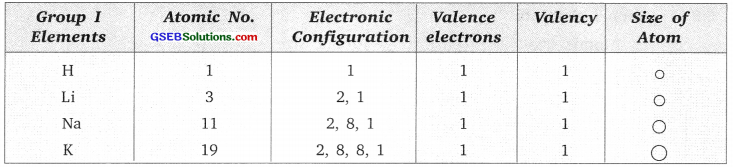Activity 5.5They contain same number of shells.

Activity 5.6
Valency of an element from its electronic configuration can be calculated by looking at the number of electrons in the outermost shell. (If the number is greater than 4, then substract 4 from the number to get valency).Activity 5.7
Atomic radii of the second period elements
Given:In decreasing order of their atomic radiiLithium has the biggest size of atom, oxygen has the smallest size of atom. On moving from Left →Right in a period the atomic size goes on decreasing.

Activity 5.8
Group I elements.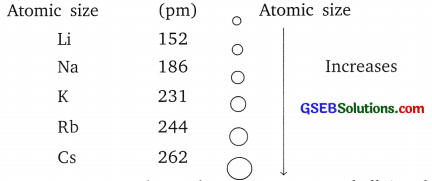Atomic size increases down the group as new shell is added as we go down the group.

Activity 5.9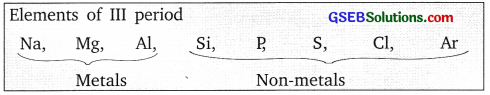Metals are towards the left-hand side of the periodic table and non-metals are towards the right hand side of the periodic table.

Activity 5.10
Tendency to lose electrons in a group increases.
Tendency to lose electrons in a period decreases.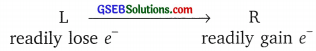Activity 5.11

• Tendency to gain electrons increases across the period.
• Tendency to gain electrons decreases down the group.

### Gujarat Board Class 10 Science Periodic Classification of Elements Textbook Questions and Answers

Question 1.
Which of the following statements is not a correct statement about the trends when going from, left to right across the periods of the Periodic Table?
(a) The elements become less metallic in nature.
(b) The number of valence electrons increases.
(c) The atoms lose their electrons more easily.
(d) The oxides become more acidic.
(c) The atoms lose their electrons more easily.Question 2.
Element X forms a chloride with the formula XCl2, which is a solid with a high melting point. X would most likely be in the same group of the Periodic Table as –
(a) Na
(b) Mg
(c) Al
(d) Si
(b) Mg.

Question 3.
Which element has –
(a) two shells, both of which are completely filled with electrons?
(b) electronic configuration 2, 8, 2?
(c) a total of three shells, with four electrons in its valence shell?
(d) a total of two shells, with three electrons in its valence shell?
(e) twice as many electrons in its second shell as in its first shell?
(a) Ne (2, 8)
(b) Mg (2, 8, 2)
(c) Si (2, 8, 4)
(d) B (2, 3)
(e) C (2, 4)

Question 4.
(a) What property do all elements in the same column of the Periodic Table as boron have in common?
(b) What property do all elements in the same column of the Periodic Table as fluorine have in common?
(a) All other elements have same valence electrons and their valency is 3.

(b) All are non-metals, they have same valence electrons i.e., 7 and their valency is 1, all of them gain electrons to form negative ions.

Question 5.
An atom has electronic configuration 2, 8, 7.
(a) What is the atomic number of this element?
(b) To which of the following elements would it be chemically similar?
(Atomic numbers are given in parentheses)
N (7) F(9) P(15) Ar (18)
(a) The atomic number of the element is 17.
(b) F(9) with electronic configuration (2, 7) will be chemically similar to the given element.Question 6.
The position of three elements A, B and C in the Periodic Table are shown below: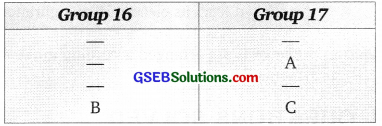(a) State whether A is a metal or non-metal.
(b) State whether C is more reactive or less reactive than A.
(c) Will C be larger or smaller in size than B?
(d) Which type of ion, cation or anion, will be formed by element A?
(a) ‘A’ is a non-metal.
(b) ‘C’ is less reactive than ‘A’
(c) ‘C’ is smaller in size than ‘B’
(d) A will form negatively charged ion Anion

Question 7.
Nitrogen (atomic number 7) and phosphorous (atomic number 15) belong to group 15 of the Periodic Table. Write the electronic configuration of these two elements. Which of these will be more electronegative? Why?
Nitrogen atomic number 7 → 2, 5.
Phosphorus atomic number 15 → 2, 8, 5
Nitrogen with two shells will be more electronegative because it can easily gain electron due to its smaller size of atom, the nuclear charge attracts the electron easily to become negative ion.

Question 8.
How does the electronic configuration of an atom relate to its position in the Modern Periodic Table?
The position of element depends upon its electronic configuration. The number of shells is equal to the period number. The valence electrons decides the group number in which it will be, elements with 1 valence electrons belong to group 1. Elements with 2 valence electrons belong to group 2.Question 9.
In the Modern Periodic Table, calcium (atomic number 20) is surrounded by elements with atomic numbers 12, 19, 21 and 38. Which of these have physical and chemical properties resembling calcium.
Ca atomic number – 20
Electronic configuration — 2, 8, 8, 2
Elements with atomic number 12 → 2, 8, 2
and atomic number 38 → 2, 8, 18, 8, 2
will resemble calcium as they all have same valence electrons and their chemical properties are also same.

Question 10.
Compare and contrast the arrangement of elements in Mendeleev’s Periodic Table and the Modern Periodic Table.
Mendeleev’s Periodic Table:

• It has 8 groups and 6 periods.
• Transition elements are not separated.
• The inert gases were not present.
• Lanthanides and Actinides were not present.
• Position of element i,e., group number and period number cannot be predicted.
• Elements are arranged according to the atomic mass.

Modem Periodic Table:

• It has 18 groups and 7 periods.
• Transition elements are given separate place.
• The inert gases are present in separate group.
• Lanthanides and Actinides are at the bottom of the Periodic Table.
• Group number and period number can be predicted from its electronic configuration.
• Elements are arranged according to the atomic number.

### Gujarat Board Class 10 Science Periodic Classification of Elements Additional Important Questions and Answers

Question 1.
Give one example of Dobereiner’s Triad.Question 2.
How many triads could Dobereiner identify from the existing elements then?
Dobemier could identify only three triads.

Question 3.
What is the limitation of Dobereiner triads?
He failed to arrange all the elements in triads having same chemical properties.

Question 4.
What was the basis of classification of elements made by Newlands?
Newlands arranged the elements in the order of increasing atomic masses.Question 5.
Give two limitations of Newlands’ law of Octaves.
Two limitations of Newlands’ law of octaves are:

• The law was applicable only upto calcium.
• In order to fit elements into his table, Newlands adjusted two elements in wrong slot, and grouped unlike elements in same groups.

Question 6.
On what basis did Mendeleev classified the element?
Mendeleev arranged the elements on the basis of their increasing atomic masses and similarity of chemical properties.

Question 7.
Which two chemical properties were considered by Mendeleev for grouping of elements?
The two chemical properties are:

• The nature of compounds formed by elements with oxygen.
• The nature of compounds formed by elements with hydrogen.

Question 8.
State Mendeleev’s Periodic Law.
The properties of elements are the Periodic function of their atomic masses.

Question 9.
Define ‘groups and periods’.
The vertical columns in a Periodic Table are called groups and the horizontal rows are called periods.

Question 10.
What is the formula of oxide and hydride of Group I elements?
Oxide formula → R2O; Hydride formula → RH.
‘R’ represents Group I element.Question 11.
Name three elements discovered later, which filled gaps left by Mendeleev for them.
Scandium, gallium and germanium.

Question 12.
What are isotopes?
Isotopes are the atoms of same element having same atomic number but different mass number.
Example: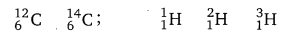Question 13.
How many groups and periods are present in the Modern Periodic Table?
Modern Periodic Table has 18 groups and 7 periods.

Question 14.
What is the location of metals and non-metals in the Modern Periodic Table?
Metals are placed on the left side and non-metals are placed on the right side of the Periodic Table.

Question 15.
State Modern Periodic Law.
‘Properties of elements are a periodic function of their atomic number’.

Question 16.
In Modern Periodic Table what is common among all the elements in a group.
All elements in same group shows same valence electrons and same chemical properties.Question 17.
Fluorine (F) atomic number = 9 and chlorine (Cl) atomic number =17 are placed in group number 17, what are the number of valence electrons present in them.
Fluorine atomic number, 9 = 2,7
Chlorine atomic number, 17 = 2, 8, 7
Both of them show 7 valence electron.

Question 18.
What is common among all the elements present in one period?
All the elements in same period show same number of shells e.g., all elements in period 3, show 3 electron shells each.

Question 19.
How many elements are present in first and second period?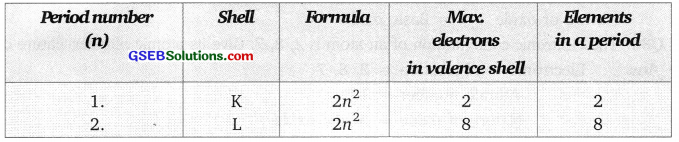Question 20.
What is atomic size?
The radius of an atom, i.e., the distance between the .centre of the nucleus and the outermost shell of an atom is called atomic size. The atomic radius is measured in picometre. (1 pm = 10-12 m)

Question 21.
What happens to the size of atom as we move from left to right in a period.
The atomic size in a period decreases as we move from left to right.Question 22.
How does the tendency to lose electrons will change in a period.
The tendency to lose electrons will decrease across a period as the effective nuclear charge acting on the valence shell electrons increases.

Question 23.
How do you think the tendency to lose electrons will change in a group?
Down the group, the effective nuclear charge experienced by valence electrons decreases, due to increase in atomic size hence they can easily lose electrons.

Question 24.
How was the anomaly in arrangement of elements in the Mendeleev’s Periodic Table removed?
When elements were arranged in the increasing order of atomic number. The anomalies of Mendeleev’s Periodic Table were removed.

Question 25.
What are noble gases/inert gases?
The element which is inactive, does not react with any other element and it has its outermost shell completely filled are called inert gases or noble gases.
Example: He, Ne, Ar, Xe.Question 26.
Name two alkali metals present in Group I.
Alkali metals are Li, Na, K.

Question 27.
An element ‘X’ belongs to II group and 2nd period. Write the atomic number and name of element.
K L ∴ Atomic Number = 4
2, 2 Element = Beryllium

Question 28.
An element ‘A has atomic number 11, name the period and group number to which it belongs.
‘A’ – atomic number = 11
Electronic configuration = K L M – 2, 8, 1
∴ Period number = Shell No. = 3
Group number = Valence electron = 1

Question 29.
An element ‘P’ belongs to group = 2 and period = 3, state whether it is a metal or non-metal and nature of its oxides.
Group 2 = Metals
Nature of oxide = Basic oxide

Question 30.
The electronic configuration of an atom is 2, 8, 7. Give its atomic number, nature of oxide.
Electronic configuration = 2, 8, 7
∴ Atomic number =17
Nature of oxide = Acidic oxideQuestion 31.
An element belongs to group 13 and period 3, name the element and give its valency.
The element is Aluminium.
The valency = 3

Question 32.
What are metalloids? Give 2 examples.
The elements which show some properties of metal and some properties of non-metal are called semi-metals or metalloids. Example – Boron, Silicon, Germanium, Arsenic.

Question 33.
An element X belongs to group 17 and element Y belongs to group 1. What type of bond will they form?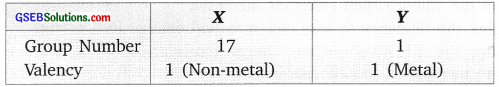Both of them will form ionic bond with the formula XY.

Question 34.
The following elements belong to same period arrange them in order.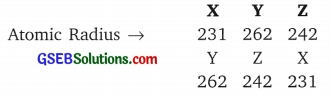The atomic size decreases in a period on going from left to right.

Question 35.
What is the valency of magnesium with atomic number 12 and nitrogen with atomic number 7?
Magnesium, atomic number =12
Electronic configuration = 2, 8, 2
∴ Valency = 2
Nitrogen, atomic number = 7
Electronic configuration = 2, 5
∴Valency = 3

Question 36.
How many shells are present in all the elements that belong to period 3?
All elements in period 3 contain 3 shells in which the electrons are distributed (K, L, M).Question 37.
What happens to the electropositive character of elements as we move from left to right of the period in the Periodic Table?
On moving from left to right in a period, the electropositive character decreases as the tendency to lose electrons decreases.

Question 38.
Fluorine, chlorine, bromine belong to same group. What is common between them?
All three elements i.e. fluorine, chlorine, bromine, have same number of valence electrons and same valency. Thus, they show similar chemical properties.

Question 39.
What are halogens? Where are they located in the Periodic Table?
Halogens are those elements which have 7 valence electrons with valency of 1 and react with metals to form salts. They are present in 17th group of the Periodic Table.

Question 40.
Atomic number of 4 elements is given below which element will belong to the group of Helium.Element Y, with atomic number 36 will belong to the same group as He.
Both are inert gas.
Atomic number of Y, 36 = 2, 8, 18, 8
Atomic number of helium, 2 = 2Question 1.
Why Mendeleev could not assign fixed position to hydrogen in the table?
(a) Hydrogen resembles alkali metal, i.e. like alkali metals it combines with halogen, oxygen and sulphur to form compounds with similar formula as alkalis.

(b) Like halogen, hydrogen also exists as diatomic molecule and combine with metal and non-metals to form covalent compounds.

Question 2.
Name the group number of the following elements, halogens, alkali metals, inert gases, hydrogen, in the Modem Periodic Table.
Halogens – group No. 17
Alkali metals – group No. 1
Inert gases – group No. 18
Hydrogen – group No. 1

Question 3.
State two characteristics of groups.
All the elements in a group have the following characteristics:
(a) All element in a group show same number of valence electrons, hence show similar properties.

(b) As we move top to bottom in a group the atomic radius goes on increasing and there is a slight gradation in properties.Question 4.
What happens to the valency of elements as we move from left to right in a Periodic Table?
As we move from left to right in a Periodic Table the valency first increases till 4 and then again decreases.
L → R
Valency in a period → 1 2 3 4 3 2 1 0

Question 5.
The number of electrons goes on increasing in the outer shell as we move from left to right in a period, why does the atomic size goes on decreasing?
In a period all elements have same number of shells. As we move from left to right in a period the number of electrons goes on increasing at the same time the number of protons also goes on increasing therefore attraction force of nucleus increases and pulls the valence electrons i.e. the outermost shell towards the nucleus and hence the size of atom goes on decreasing.

Question 6.
What happens to the metallic character as we move from top to bottom in a group?
The metallic character increases as we move from top to bottom as the tendency to lose electrons increases.

Question 7.
What happens to the non-metallic character as we move from top to bottom in a group?
The non-metallic character decreases as we move from top to bottom in a group as the tendency to gain electrons decreases down the group.

Question 8.
The atomic number of ‘X’ is 17. Predict its (a) valency, (b) formula of halide, (c) type of ion formed, (d) reactivity with respect to the other members of same group.
‘X’ has atomic number 17
∴ Electronic configuration → 2, 8, 7
(a) Valency = 1
(b) Formula of halide = HX
(c) Type of ion formed = X, Negative ion (Anion).
(d) Reactivity = Most reactive among those elements which lie below X in a group.Question 9.
Given below are 4 elements W, X, Y and Z the atomic numbers are 9, 10, 16, 17.
Predict the following:
(a) Two elements lying in same group.
(b) Elements in second period.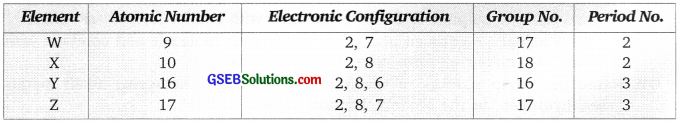(a) Two elements in same group – W and Z
(b) Elements in second period – W and X

Question 10.
Write all the elements present in third period of the Periodic Table and give their electronic configuration.
Elements of third period are:Question 11.
How does electronic configuration helps us to locate the position of element in the Periodic Table?
The electronic configuration of an atom conveys the valence electrons and number of shells. Valence electrons helps in detecting the group number. Number of shells in an atom tells the period to which it belongs.

Question 12.
What are the merits of Mendeleev’s Periodic Table?
Merits of Mendeleev’s Periodic Table are:
1. Mendeleev left some gaps in his table. Predicted the chemical properties of these 3 elements which were discovered later and had same properties as predicted by Mendeleev, they were gallium, germanium and scandium.

2. He arranged the elements very systematically in periods and groups.Question 13.
Why does the reactivity of metals increases and that of non-metals decreases as we move down the group?
Reactivity of metals depends on the tendency to lose electrons. If the atomic size increases, the valence electrons are easily removed thereby forming positive ion. In case of metals the atomic size increases as we move down the table. Reactivity of non-metals depends on the tendency to gain electrons. As we move down the group, the tendency to gain electrons decreases because the atomic size increases, the nuclear force decreases.

Question 14.
List the elements present in 2nd period. Write their atomic number and electronic configuration.Question 15.
For the following given elements predict the;
(a) Valency
(b) Period number
(c) Group number Na (11), Al(13), Cl(17), K(19)Question 16.
Elements of group 1 are given below with their atomic number: Li (3) Na (11) K (19)
Give the order of their (a) atomic size. (b) reactivity.(a) The atomic size goes on increasing as new shell is added in each element as we move from top to bottom. So it is Li < Na < K.

(b) The reactivity increases as it is easy to lose electrons if the size of atom increases due to decrease in nuclear force. So, it is K < Na < Li.

Question 17.
Lithium, sodium and potassium belong to same group called alkali metals. Why?
Lithium, sodium and potassium have same valence electron i.e. 1, hence they belong to same group. The group is called alkali metals group because all these elements form oxides which dissolve in water to form alkali.Question 18.
Carbon with atomic number 6 and silicon with atomic number 14 belong to same group although carbon is non-metal and silicon is semi-metal. Comment.
Silicon with electronic configuration 2, 8, 4 have three shells while carbon with electronic configuration 2, 4 have two shells. Thus, silicon is bigger in size and have lower nuclear attraction than carbon which leads to easier removal of electron or metallic character from silicon than carbon.

Question 19.
The position of 3 elements A, B and C in the Periodic Table is shown below:Giving reasons, explain the following:
(a) Element A is a non-metal.
(b) Atom of element C has larger size than A.
(c) Element B has a valency of 1.
(a) A belongs to VII group, has 7 valence electron, it can gain 1 electron to become stable. So it is a non-metal as it forms negative ion.

(b) ‘C’ has 3 shells A’ has 2 shells so C is bigger than A.

(c) ‘B’ has one valence electron, it can’lose this electron to become stable. So its valency is 1.

Question 20.
The elements of the third period of the Periodic Table are given below:(a) Which atom is bigger – Na or Mg? Why?
(b) Identify the most – (i) Metallic and (ii) Non-metallic element in period 3.
(a) Na atom is bigger in size this is because as we move from Na to Cl, the atomic number goes on increasing and the nuclear charge also increases. It pulls/attract the valence electrons at the centre and thus the atomic size decreases.

(b) (i) Most metallic – Na
(ii) Most non-metallic – ClQuestion 1.
The atomic number of element X is 17 predict its
(a) Physical state.
(b) Name of element.
(c) Formula of its compound with hydrogen
(d) Metal or Non-metal.
(e) Formula of its molecule.
Atomic number of X = 17.
Electronic configuration = 2, 8, 7
(a) Physical state → Gas
(b) Chlorine
(c) HCl
(d) Non-metal
(e) Cl2

Question 2.
Two elements A and B belong to group 1 and 2 respectively in the same period. Compare them with respect to:
(a) Valency
(b) Size of atom
(c) Formula of oxide
(d) Nature of oxide
(e) Metallic character
(a) Valency A → 1, B → 2
(b) Size of atom → A is bigger atom than B.
(c) Formula of oxide → A2O, BO
(d) Nature → Basic
(e) Metallic character → A is more metallic than B.Question 3.
Give all characteristics of group.
Characteristics of a group:
(a) Valence electrons → All elements show same valence electrons in a group.
(b) Valency → Valency of all the elements remains the same in a group.
(c) Atomic size → The atomic size goes on increasing down the group.
(d) Metallic character → In case of metals the metallic character increases down the group.
(e) Non-metallic character → In case of non-metals the non-metallic character decreases down the group.

Question 4.
Give the characteristics of a period.
In a period as we go from left to right:
(a) Valence electrons → Goes on increasing (1, 2, 3, 4, 5, 6, 7, 8.)
(b) Valency → Valency first increases up to 4 and then decreases to zero (1, 2, 3, 4, 3, 2, 1, 0).
(c) Size of atom → Size of atom goes on decreasing
(d) Metallic character → Decreases
(e) Non-metallic character → Increases

Question 5.
You are given some descriptions about five different elements. Identify these elements to place them in the Modern Periodic Table.
(a) Essential for breathing and burning.
(b) Inactive, two electrons in the outermost shell.
(c) Atom has same number of protons, electrons and neutrons, used in fertilizer industry.
(d) Number of neutrons, protons are same used in building our bones.
(e) This element form the hardest naturally occurring substance as allotrope.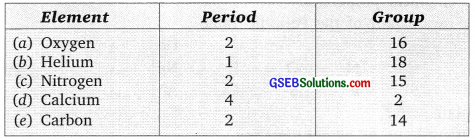Question 6.
Name the following elements
(a) Two shells, both of which are completely filled.
(b) Three shells with 2 valence electrons.
(c) Group 1, two shells.
(d) Group 17, period 3.
(e) Metal, with valency 3 group number 13 period 3.
(a) Two shells → K L
Filled → 2, 8
Atomic number → ∴ Element = Neon

(b) Three shells → K, L, M
2 Valence electron → 2, 8, 2
Atomic number → 12 ∴ Element = Magnesium

(c) Group 1
2 shells → K L
Electronic configuration → 2,
1 Atomic number → 3 ∴ Element = lithium

(d) Group 17 and Period → 3, K L M
Electronic configuration → 2, 8, 7
Atomic number → 17 ∴ Element = chlorine

(e) Group → 13; Valency → 3
Period → 3 K L M
Electronic configuration → 2, 8, 3
∴ Atomic number → 13, ∴ Element = Aluminium

Practical Based Questions Solved (Solved)

Question 1.(a) Which atom is bigger, Na pr Mg? Why?
(b) Identify the most metallic and most non-metallic element in period 3.
(a) Sodium is bigger is size than magnesium because the effective nuclear charge of sodium is less than magnesium and the electrons in the outer shell are more attracted to the center of the magnesium atom.

(b) The most metallic element is Na as it can readily loose electron to form positively charged ion. Chlorine is the most nonmetallic element as it can readily gain electrons to from negatively charged ion.Question 2.
For each of the following, give the name of an element from Period 3 (sodium to argon), which matches the description.
(a) an element which is gaseous at room temperature and pressure
(b) an element that is added to water to kill bacteria
(c) an element that forms a basic oxide of the type XO
(d) an element that forms an amphoteric oxide
(e) an element that reacts vigorously with cold water to produce hydrogen.
(a) Chlorine
(b) Chlorine
(c) Magnesium
(d) Aluminium
(e) Sodium

Question 3.
The table given below shows four elements, named P, Q, R and S. Complete the table: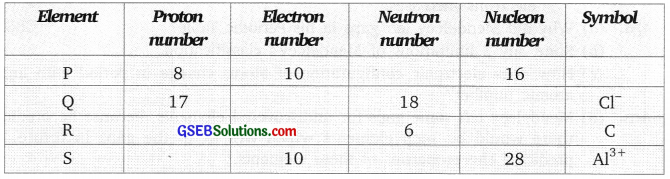Nucleon number is the number of protons and neutrons in a nucleus of an atom.

Question 4.
Rubidium, Rb, is a Group I element. It has similar physical and chemical properties to the other elements in Group I
(a) When rubidium is added to cold water a reaction occurs. Suggest two observations that would be made when rubidium is added to cold water.
(b) Write a chemical equation for the reaction between rubidium and water.
(c) Suggest one safety measure that should be used when rubidium is added to cold water.
(a) The reaction is very vigorous, flames can be seen, hissing sound can be produced

(b) 2Rb +2H2O → 2RbOH + H2

(c) The reaction is highly exothermic and the splash of hot water or rubidium may fall on body hence stay at distance, wear safety glasses, lab coat and hand gloves.

Question 5.
Aluminium metal is widely used in the making of cooking utensils like pans, cookers etc. Suggest the property of aluminium that makes it suitable for this use.